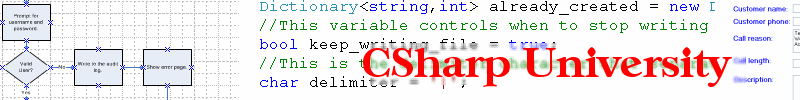"Join my free Amazing ASP.NET newsletter"
It's safe and easy to signup. You get:
• Notified anytime a new article comes out
• Technical step-by-step tutorials on all important ASP.NET topics
• Expert guidance so you can reach unlimited ASP.NET success

This exercise is a continuation of the Lab2 website project that we created in other lessons in the Conditionals and Loops category.  Now we are going to move on and discuss loops.  In addition to making decisions, C# offers constructs to assist with repetitive tasks and logic that is redundant through the use of loop keywords.  The first loop type commonly used is the “for” loop.

Create a new web form in the Lab2 project and leave the name as Default3.aspx.  Goto the Design view for the form and drag a Button and TextBox as we did in similar prior exercises.  Change the Text property of the Button to say “for loop” and then create the event handler method.  In the event handler put the following:

```

for (int i = 1; i <= 5; i++)
{
TextBox1.Text += i.ToString();
}
```

Let’s take a look at the loop step by step.  First notice the keyword “for” which lets C# know that you want to keep repeating whatever is inside the open and closed curly braces {  } that immediately follow the “for” statement line.  The first part of the for loop is called the initialization statement, in our case this is int i = 1;  The second part of the for loop is the termination condition, in our case i <= 5;  The third part of the for loop is the increment statement, in our casee i++.

In plain English, here is how C# interprets the for loop in the example above.  First C# will execute the initialization statement before the loop runs for the first time.  So it will create a variable called i and assign a value of 1.  This happens only one time before the loop executes.

Next C# will run the termination condition to see if it is true.  If the termination condition is true, C# will keep on looping and executing all the code inside the curly braces.  If the termination condition is false, C# will no longer execute the loop.

At the end of each loop iteration (or cycle), after the code in the curly braces has been executed, C# executes the increment statement of the for loop which is i++ in our example.  In C# the ++ operator adds one to the variable that prefixes the operator.  It is the same as doing i = i + 1;  So think of it as shorthand.  Here is the order of operations in the for loop in our example:

Initialize the variable i
Is i <= 5? Yes, so execute the loop
Display the number 1 in the TextBox
Increment i by 1, i now is 2
Is i <= 5? Yes, so execute the loop
Display the number 2 in the TextBox
Increment i by 1, i now is 3
Is i <= 5? Yes, so execute the loop
Display the number 3 in the TextBox
Increment i by 1, i now is 4
Is i <= 5? Yes, so execute the loop
Display the number 4 in the TextBox
Increment i by 1, i now is 5
Is i <= 5? Yes, so execute the loop
Display the number 5 in the TextBox
Increment i by 1, i now is 6
Is i <= 5? No, so terminate the loop

A lot of C# programmers tend to start their loops with zero instead of 1 for various reasons.  I want you to see how the loop above could have been written slightly differently but still execute the same number of times:

```

for (int i = 0; i < 5; i++)
{
TextBox1.Text += i.ToString();
}
```

The loop variable i (also known as the loop index variable) would have been assigned values of 0, 1, 2, 3, 4 and finally 5 at which time the loop would terminate.  This means that the loop would run a total of 5 iterations, just like in the prior example where I had started with i = 1 instead;

In my experience, for loops are generally useful if you know roughly how many times you need to execute a block of code.  For example, if you need to loop through an Array and pull items that are in that Array, the Array has a property called Length which can be used in the for loop to specify how many iterations to run.

I have also found it good practice not to change the value of the loop index variable inside of the loop body.  In other words, don’t change the value of i inside your loop.  This can create unwanted conditions that are difficult to debug and generally should be avoided.